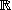# Genspace --- Introduction ---

This module is an exercise of linear algebra, on the generation of the vector spacen by a given set of vectors.

The exercise first gives you the vectors (randomly generated), and automatically computes the rank of the matrix formed by these vectors. You have to determine, according to these data, whether the vectors generate the whole space, and give the reason for your reply.

A second question will be asked if the exercise is in an advanced mode. This second question depends on the first reply: if you think that the vectors generate the space, you will be presented another vector, and should express it as a linear combination of the given generator vectors. Otherwise, you should find a vector in the space which is not in the subspace generated by the given vectors.

You may go to work on the exercise, by choosing the level of difficulty:

1  2  3  4  5  6

Other exercises on: vector space   matrices   Basis   Vectors   linear algebra

In order to access WIMS services, you need a browser supporting forms. In order to test the browser you are using, please type the word wims here: and press Enter''.